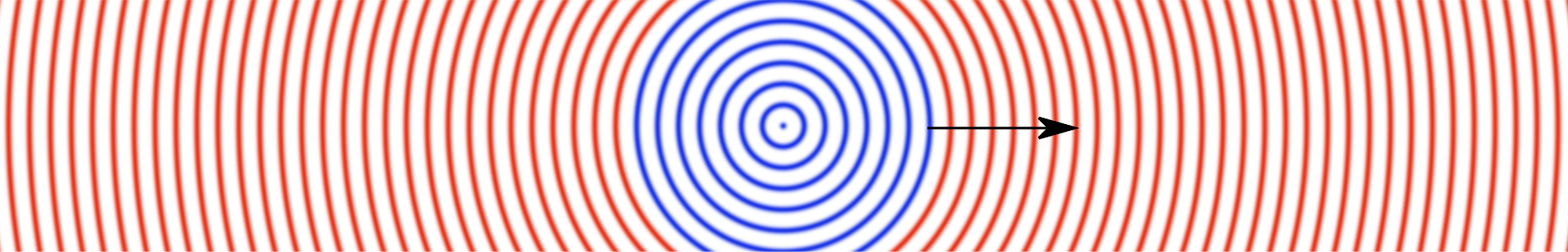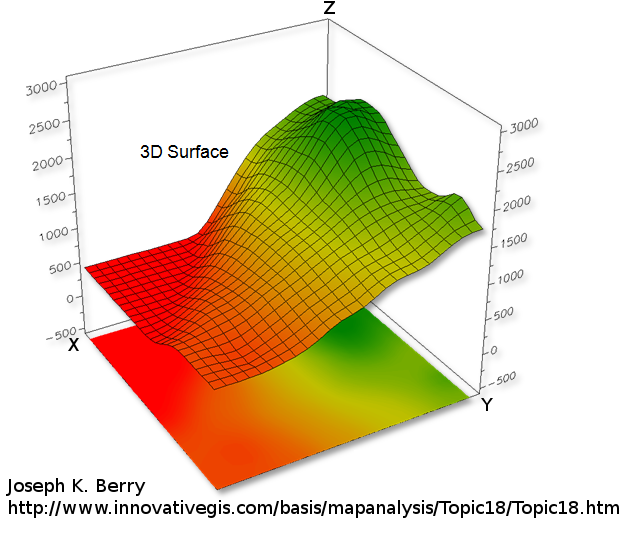# Find a tetrahedron circumcenter# Definition: Laplacian Matrix for triangle meshesDefinition of the Laplacian matrix and Laplace operator on a triangular mesh. I give an in depth explanation here.

# Normal to an implicit surfaceCheat sheet to differentiate expressions with the $$\nabla$$ operator to compute gradients of various functions.

# Contour lines## Introduction

To represent a 2D function $$z = f(x,y)$$ one can draw a 3D surface. The higher the $$z$$ value the higher the point on the surface (see above).

# Curvature of a triangle mesh, definition and computation.Defining and giving the formula to compute the curvature over a triangle mesh at some vertices.

• 1